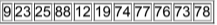×
Get Full Access to Elementary Statistics - 12 Edition - Chapter 3.2 - Problem 19bsc
Get Full Access to Elementary Statistics - 12 Edition - Chapter 3.2 - Problem 19bsc

×

# In Exercises, find the (a)mean, (b) median, | Ch 3.2 - 19BSCISBN: 9780321836960 18

## Solution for problem 19BSC Chapter 3.2

Elementary Statistics | 12th Edition

• Textbook Solutions
• 2901 Step-by-step solutions solved by professors and subject experts
• Get 24/7 help from StudySoup virtual teaching assistantsElementary Statistics | 12th Edition

4 5 1 362 Reviews
20
1
Problem 19BSC

Saints in Super Bowl Listed below are the numbers on the jerseys of the starting lineup for the New Orleans Saints when they recently won their first Super Bowl football game. What do the measures of center tell us about the team? Does it make sense to compute the measures of center for these data?Step-by-Step Solution:

Step 1 of 1

(a) The "Mean" is computed by adding all of the numbers in the data together and dividing by the number elements contained in the data set.

Mean == 50.36

(b) The "Median" of a data set is dependant on whether the number of elements in the data set is odd or even. First reorder the data set from the smallest to the largest then if the number of elements are odd, then the Median is the element in the middle of the data set. If the number of elements are even, then the Median is the average of the two middle terms.

 9 12 19 23 25 73 74 76 77 78 88

Median = 73

(c) The "Mode" for a data set is the element that occurs the most often. It is not uncommon for a data set to have more than one mode. This happens when two or more elements occur with equal frequency in the data set. A data set with two modes is called bimodal. A data set with three modes is called trimodal.

Since there is no repeated terms in the data. Hence there is no mode.

(d) In statistics, the mid-range or mid-extreme of a set of statistical data values is the arithmetic mean of the maximum and minimum values in a data set, defined as

Mid range =48.5

Step 2 of 1

##### ISBN: 9780321836960

The answer to “?Saints in Super Bowl Listed below are the numbers on the jerseys of the starting lineup for the New Orleans Saints when they recently won their first Super Bowl football game. What do the measures of center tell us about the team? Does it make sense to compute the measures of center for these data?” is broken down into a number of easy to follow steps, and 55 words. This full solution covers the following key subjects: bowl, super, Saints, measures, given. This expansive textbook survival guide covers 121 chapters, and 3629 solutions. Elementary Statistics was written by and is associated to the ISBN: 9780321836960. Since the solution to 19BSC from 3.2 chapter was answered, more than 381 students have viewed the full step-by-step answer. This textbook survival guide was created for the textbook: Elementary Statistics, edition: 12. The full step-by-step solution to problem: 19BSC from chapter: 3.2 was answered by , our top Statistics solution expert on 03/15/17, 10:30PM.

Unlock Textbook Solution